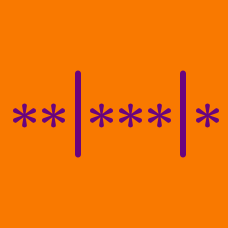Probability

# Identical Objects into Identical Bins

How many ways are there to put 7 identical marbles into non-empty groups?

How many ways are there to put 14 identical oranges into 6 non-empty groups?

Which of the following is equal to the number of distributions of 28 identical objects into exactly 9 identical non-empty boxes?

How many ways are there to organize 3 identical red balls and 4 identical blue balls into 3 identical containers such that no container is empty?

How many ways are there to put 10 identical coins into 5 non-empty groups?

×